Ch 12. Infinite Sequences and Series Multimedia Engineering Math Sequencesand Series Integral andComparison Alt. Series &Abs. Conv. PowerSeries Taylor, Mac. &Binomial
 Chapter 1. Limits 2. Derivatives I 3. Derivatives II 4. Mean Value 5. Curve Sketching 6. Integrals 7. Inverse Functions 8. Integration Tech. 9. Integrate App. 10. Parametric Eqs. 11. Polar Coord. 12. Series Appendix Basic Math Units Search eBooks Dynamics Fluids Math Mechanics Statics Thermodynamics Author(s): Hengzhong Wen Chean Chin Ngo Meirong Huang Kurt Gramoll ©Kurt GramollMATHEMATICS - CASE STUDY Introduction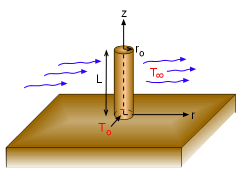A Circular Fin Protruding from a Hot Wall Fins are often used as a medium to increase heat transfer. Joseph, an engineer, is interested in using a circular fin with radius ro and length L to cool a hot wall. The wall temperature at the base of protruding fin is To. The fin is cooled by a fluid stream at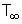. Based on constant thermal conductivity and an infinite heat transfer coefficient assumption, the two-dimensional steady-state temperature distribution of the fin is,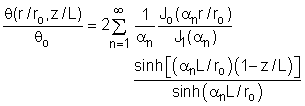where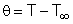,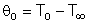and αn's are the positive roots of Jo(αn) = 0 for n = 1, 2, 3, ... Joseph would like to find the temperature value at a particular point on the fin. Questions Determine the value of θ/θo for r/ro = 0.25, L/ro = 5, and z/L = 0.5. Approach Jo(αn), the Bessel function, is zero when α1 = 2.405, α2 = 5.520, α3 = 8.654, and α4 = 11.792 Determine the values of the Bessel functions Jo(αnr/ro) and J1(αn) based on the power series expansion with at least five terms (Note: This is the approach only if tables and graphs of Bessel funtion values are not available).